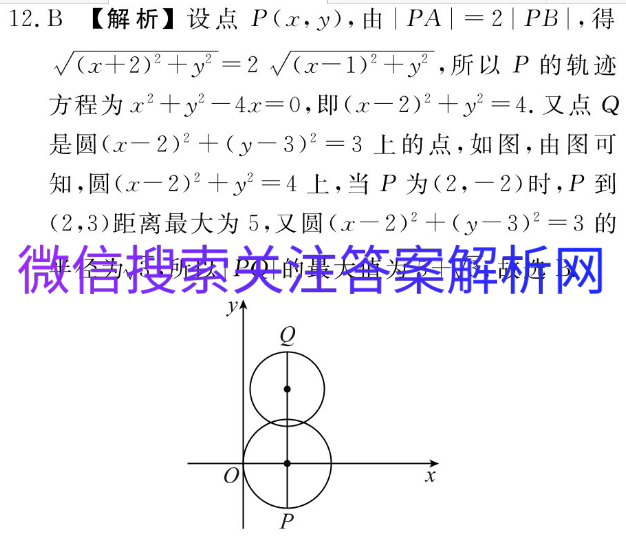# 炎德英才大联考官网答案在

2021-01-09 21:5512.B【解析】设点P(x,y),由|PA|=2|PB|,得√(x+2)2+y2=2√(x-1)2+y2,所以P的轨迹方程为x2+y2-4x=0,即(x-2)2+y2=4.又点Q是圆(x-2)2+(y-3)2=3上的点,如图,由图可知,圆(x-2)2+y2=4上,当P为(2,-2)时,P到(2,3)距离最大为5,又圆(x-2)2+(y-3)2=3的半径为√3,所以|PQ的最大值为5+√3.故选B.y↑QOP